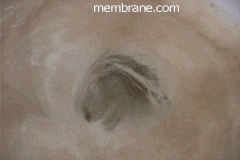# The Cron VortexThe Initiation of a Revolutionary Revelation## The Universal Theorem contains elements from:Einstein's Theory of Relativity / Space-time Continuum, Vortex Physics, Chaos Theory and The Laws of Thermodynamics Abstract Earth is in a space-time continuum similar to the vortex of a flushing toilet.

The Universal Theorem contains elements from :

The combination of said elements form a system. The interaction between the system and matter is commonly called time.

Toilet Flushing (MP4 Video)

A team of researchers is gathering to conduct experiments in this field. Members include a physicist, engineer, mathematician, statistician, logician, and philosopher. If you have anything to contribute, please click here.

## Example Problem Where Earth is in a Vortex

if acceleration = velocity/time
and v = speed of light = 2.9979*10^8 m/s
and for the sake of argument we are accelerating at pi (rounded to 15 decimal places) m/s^2

then time = 95426120.77903860 seconds (rounded to 16 significant digits)
= 1104.468990498130 days
= 3.025942439720910 years = roughly 3 years + 9.5 days as relative to the rotation and revolution of the planet earth.

a couple other fundamental physical constants to contemplate:
permitivity of free space = 8.854*10^(-12) C^2/(N*m)
permeability of free space = 4(pi)*10^(-7) Wb/(A*m)

## Practical Application Of Cron Vortex Click Back to the outer edges.

© The Philadelphia Spirit Experiment Publishing Company These graphics, images, text copy, sights or sounds may not be used without expressed written consent.